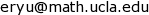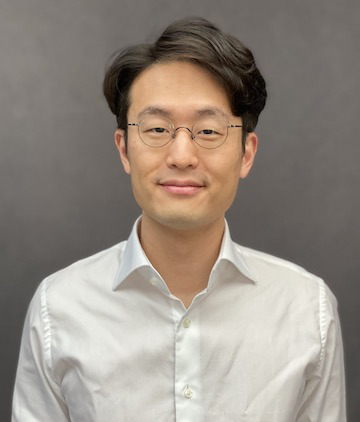## Infinitely Large Neural Networks, Spring 2022

This is the public course website for Topics in Applied Mathematics: Infinitely Large Neural Networks (응용수학특강: 심층신경망의 수학적 극한), 3341.751, Spring 2022. We will use eTL as a secondary website.

## Homework

This class will have written homework assignments. (No programming.) Submit completed assignments through eTL.

## Lecture Plans

• [Weeks 1–2] Universal approximation theory: Wide neural networks
• [Weeks 3–4] Kernel methods
• [Week 5] Random feature learning
• [Week 6] Continuous-time analysis of gradient descent
• [Week 7] Gaussian processes and deep neural networks as Gaussian processes
• [Week 8] Neural tangent kernel of infinitely wide neural networks
• [Weeks 9–10] Mean-field analysis of infinitely wide neural networks
• [Week 11] Universal approximation theory: Deep neural networks
• [Week 12] Neural ODE and equilibrium models
• [Week 13] Trainability of infinitely deep neural networks

## Course Description

Although the empirical success of deep learning is apparent, a theoretical explanation of this phenomenon remains elusive. In this course, we study the recent deep learning theory that aims to address this question.

We start with the representation power of wide neural networks. The classical theory shows that wide 2-layer neural networks are universal approximators, i.e., they can approximate arbitrary functions. However, this line of work does not address whether one can find such approximations using SGD or any practical algorithm.

Next, we study kernel methods and reproducing kernel Hilbert spaces (RKHS). While the classical "kernel trick" is not directly used in modern deep learning, the RKHS theory will serve as the functional analytical language for our later discussion of the dynamics of functions.

Next, using RKHS theory, we study random feature learning, an implementable non-deep learning method with provable $\mathcal{O}(1/\sqrt{N})$ guarantees. Random feature learning serves two important purposes: (i) A simple baseline for deep learning algorithms to surpass theoretically (often not accomplished) (ii) A stepping stone to the NTK theory.

Next, we study gradient flow, a continuous-time model of (stochastic) gradient descent. Deep learning theory often relies on two simplifications (i) that the network is infinitely large (ii) the neural network is trained with gradient flow rather than SGD. We study when and in what sense this approximation is accurate and what benefit this simplification brings.

Next, we study Gaussian processes and how randomly initialized deep neural networks behave as Gaussian processes at initialization (before training).

Next, we study the neural tangent kernel (NTK) theory. Using the theory of kernels and Gaussian processes, we establish a CLT-like limit of infinitely wide neural networks of fixed depth and establish trainability guarantees.

Next, we study the mean-field (MF) theory of deep neural networks. Using the theory of partial differential equations, we establish an LLN-like limit of infinitely wide neural networks of depth 2 (and $\ge 3$) and establish trainability guarantees.

Next, we shift the attention from infinitely wide neural networks to infinitely deep ones. We show that deep neural networks are also universal approximators.

Next, we study Neural ODE and equilibrium models, which be viewed as neural networks with continuous (infinite) depth. We define the infinite-depth limits and obtain the continuous-depth counterpart to backprop, which allows one to practically train these models using well-established ODE solvers. However, these models by themselves do not have trainability guarantees.

Finally, we study the trainability of infinitely deep neural networks. This requires combining mean-field theory with continuous-depth models.

## References

• Universal approximation theory: Wide neural networks
• G. Cybenko, Approximation by superpositions of a sigmoidal function, Mathematics of Control, Signals, and Systems, 1989.
• A. Pinkus. Approximation theory of the mlp model in neural networks. Acta Numerica, 1999.
• K. Hornik. Approximation capabilities of multilayer feedforward networks. Neural Networks, 1991.
• A. R. Barron. Universal approximation bounds for superpositions of a sigmoidal function. IEEE Transactions on Information Theory, 1993.
• Kernel methods
• Random feature learning
• A. Rahimi and B. Recht, Random features for large-scale kernel machines, NeurIPS, 2007.
• A. Rahimi and B. Recht, Weighted sums of random kitchen sinks: Replacing minimization with randomization in learning, NeurIPS, 2008.
• A. Rahimi and B. Recht, Uniform approximation of functions with random bases. Allerton Conference, 2008.
• Continuous-time analysis of gradient descent
• No external reference.
• Gaussian processes and deep neural networks as Gaussian processes
• J. Lee, Y. Bahri, R. Novak, S. S. Schoenholz, J. Pennington, J. Sohl-Dickstein, Deep Neural Networks as Gaussian Processes, ICLR, 2018.
• Neural tangent kernel
• A. Jacot, F. Gabriel, C. Hongler, Neural Tangent Kernel: Convergence and Generalization in Neural Networks, NeurIPS, 2018.
• S. Arora, S. S. Du, W. Hu, Z. Li, R. Salakhutdinov, R. Wang, On Exact Computation with an Infinitely Wide Neural Net, NeurIPS, 2019.
• J. Lee, L. Xiao, S. S. Schoenholz, Y. Bahri, R. Novak, J. Sohl-Dickstein, and J. Pennington, Wide Neural Networks of Any Depth Evolve as Linear Models Under Gradient Descent, NeurIPS, 2019.
• Mean-field analysis of infinitely wide neural networks
• L. Chizat and F. Bach, On the global convergence of gradient descent for over-parameterized models using optimal transport. NeurIPS, 2018.
• S. Mei, A. Montanari, and P.-M. Nguyen, A mean field view of the landscape of two-layer neural networks. Proceedings of the National Academy of Sciences, 2018.
• H. T. Pham and P.-M. Nguyen, Global Convergence of Three-layer Neural Networks in the Mean Field Regime, ICLR, 2021.
• Universal approximation theory: Deep neural networks
• P. Kidger and T. Lyons, Universal Approximation with Deep Narrow Networks, COLT, 2020.
• S. Park, C. Yun, J. Lee, and J. Shin, Minimum Width for Universal Approximation, ICLR, 2021.
• Neural ODE and equilibrium models
• R. T. Q. Chen, Y. Rubanova, J. Bettencourt, and D. K. Duvenaud, Neural Ordinary Differential Equations, NeurIPS, 2018.
• Trainability of infinitely deep neural networks
• Y. Lu, C. Ma, Y. Lu, J. Lu, L. Ying, A Mean Field Analysis Of Deep ResNet And Beyond: Towards Provably Optimization Via Overparameterization From Depth, ICML, 2020.
• Z. Ding, S. Chen, Q. Li, S. Wright, Overparameterization of deep ResNet: zero loss and mean-field analysis, arXiv, 2021.

## Course Information

### Instructor

Ernest K. Ryu, 27-205,### Lectures

Tuesdays and Thursdays 3:30–4:45pm in hybrid format, at 500-L306 and over Zoom. Lectures will be delivered on the blackboard. Live (in-person or online) attendance is required. Zoom link and the password are available on eTL. We will try to provide typed lecture notes.

### Exams

This class will have in-person midterm and final exams.

• Midterm exam: Thursday, 04/28, 5:00–8:00pm, location TBD.
• Final exam: Tuesday, 06/21, 3:30–6:30pm, location 500-L306.

• Functional analysis at the level of the Hahn–Banach theorem and $L^p$ spaces.